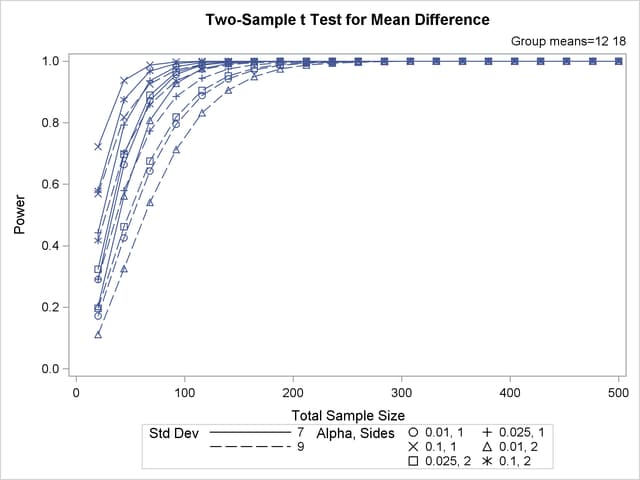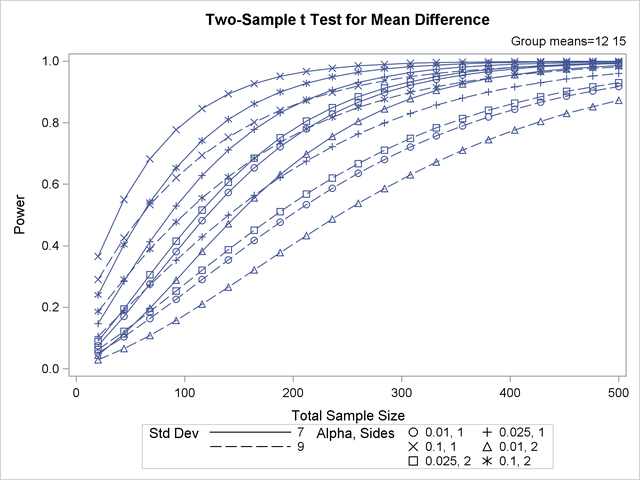### Linking Plot Features to Analysis Parameters

You can use the VARY option in the PLOT statement to specify which of the following features you want to associate with analysis parameters.

• line style

• plotting symbol

• color

• panel

You can specify mappings between each of these features and one or more analysis parameters, or you can simply choose a subset of these features to use (and rely on default settings to associate these features with multiple-valued analysis parameters).

Suppose you supplement the sample size analysis in Output 70.8.5 to include three values of alpha, by using the following statements:

```proc power plotonly;
twosamplemeans test=diff
groupmeans   = 12 | 15 18
stddev       = 7 9
alpha        = 0.01 0.025 0.1
power        = .
ntotal       = 100;
plot x=n min=20 max=500;
run;
```

The defaults for the VARY option in the PLOT statement specify line style varying by the ALPHA= parameter, plotting symbol varying by the GROUPMEANS= parameter, panel varying by the STDDEV= parameter, and color remaining constant. The resulting plot, consisting of two panels, is shown in Output 70.8.14.

Output 70.8.14 Plot with Default VARY Settings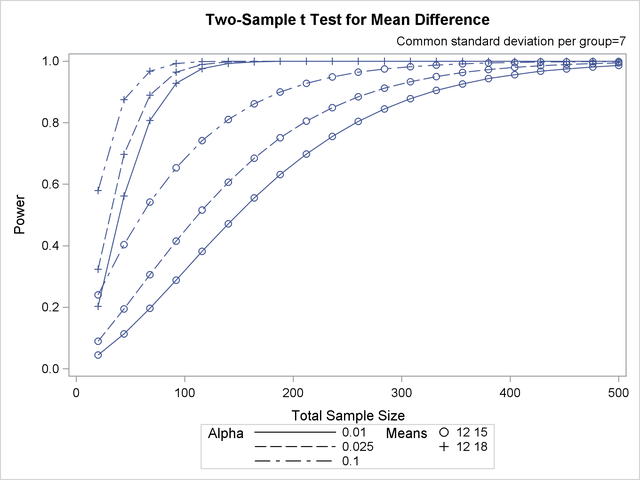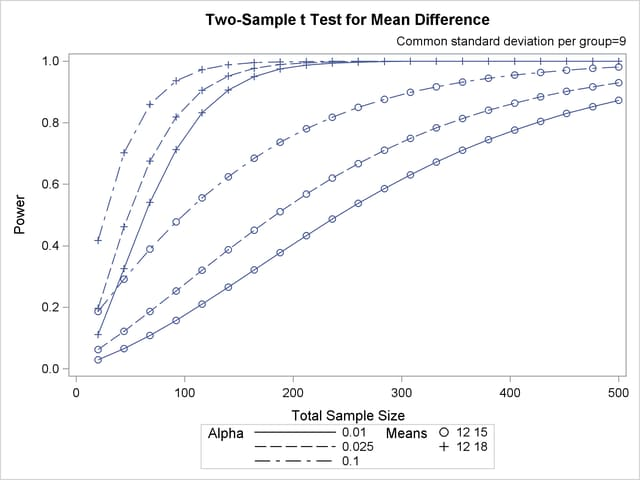Suppose you want to produce a plot with only one panel that varies color in addition to line style and plotting symbol. Include the LINESTYLE, SYMBOL, and COLOR keywords in the VARY option in the PLOT statement, as follows, to produce the plot in Output 70.8.15:

```   plot x=n min=20 max=500
vary (linestyle, symbol, color);
```

Output 70.8.15 Plot with Varying Color Instead of Panel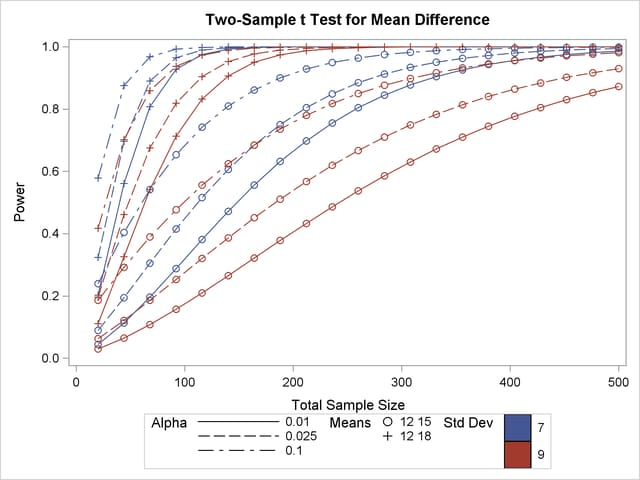Finally, suppose you want to specify which features are used and which analysis parameters they are linked to. The following PLOT statement produces a two-panel plot (shown in Output 70.8.16) in which line style varies by standard deviation, plotting symbol varies by both alpha and sides, and panel varies by means:

```   plot x=n min=20 max=500
vary (linestyle by stddev,
symbol by alpha sides,
panel by groupmeans);
```

Output 70.8.16 Plot with Features Explicitly Linked to Parameters